A 3 volt battery with negligible internal resistance is connected in a circuit as shown in the figure. The current I, in the circuit will be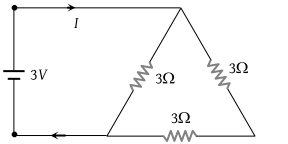(1) 1/3 A

(2) 1 A

(3) 1.5 A

(4) 2 A

Concept Questions :-

Combination of resistors
High Yielding Test Series + Question Bank - NEET 2020

Difficulty Level:

Find the equivalent resistance between the points a and b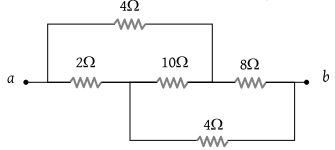(1) 2 Ω

(2) 4 Ω

(3) 8 Ω

(4) 16 Ω

Concept Questions :-

Wheatstone bridge
High Yielding Test Series + Question Bank - NEET 2020

Difficulty Level:

The potential difference between point A & B is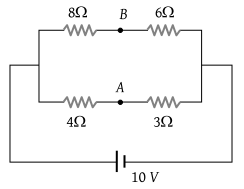(1) $\frac{20}{7}\text{\hspace{0.17em}\hspace{0.17em}}V$

(2) $\frac{40}{7}\text{\hspace{0.17em}\hspace{0.17em}}V$

(3) $\frac{10}{7}\text{\hspace{0.17em}\hspace{0.17em}}V$

(4) 0

Concept Questions :-

Wheatstone bridge
High Yielding Test Series + Question Bank - NEET 2020

Difficulty Level:

In the circuit shown below, The reading of the voltmeter V is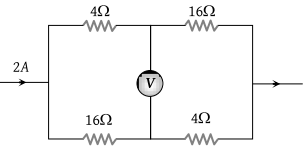(1) 12 V

(2) 8 V

(3) 20 V

(4) 16 V

Concept Questions :-

Wheatstone bridge
High Yielding Test Series + Question Bank - NEET 2020

Difficulty Level:

In the circuit shown in the adjoining figure, the current between B and D is zero, the unknown resistance is of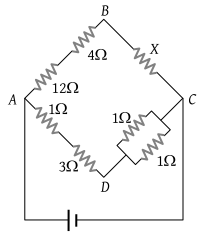(1) 4 Ω

(2) 2 Ω

(3) 3 Ω

(4) em.f. of a cell is required to find the value of X

Concept Questions :-

Wheatstone bridge
High Yielding Test Series + Question Bank - NEET 2020

Difficulty Level:

In the circuit shown in the figure, the current flowing in 2 Ω resistance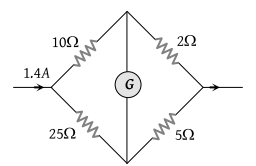(1) 1.4 A

(2) 1.2 A

(3) 0.4 A

(4) 1.0 A

Concept Questions :-

Wheatstone bridge
High Yielding Test Series + Question Bank - NEET 2020

Difficulty Level:

Five resistors are connected as shown in the diagram. The equivalent resistance between A and B is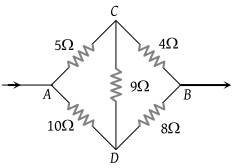(1) 6 ohm

(2) 9 ohm

(3) 12 ohm

(4) 15 ohm

Concept Questions :-

Wheatstone bridge
High Yielding Test Series + Question Bank - NEET 2020

Difficulty Level:

In the figure given the value of X resistance will be, when the p.d. between B and D is zero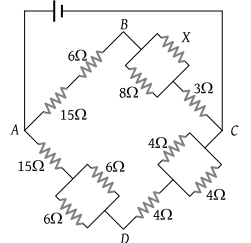(1) 4 ohm

(2) 6 ohm

(3) 8 ohm

(4) 9 ohm

Concept Questions :-

Wheatstone bridge
High Yielding Test Series + Question Bank - NEET 2020

Difficulty Level:

The effective resistance between points A and B is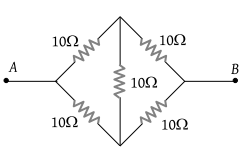(1) 10 Ω

(2) 20 Ω

(3) 40 Ω

(4) None of the above three values

Concept Questions :-

Wheatstone bridge
High Yielding Test Series + Question Bank - NEET 2020

Difficulty Level:

Five resistors of given values are connected together as shown in the figure. The current in the arm BD will be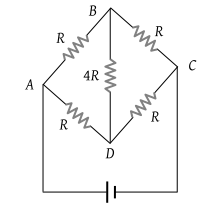(1) Half the current in the arm ABC

(2) Zero

(3) Twice the current in the arm ABC

(4) Four times the current in the arm ABC

Concept Questions :-

Wheatstone bridge